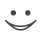# Get these flashcards, study & pass exams. For free! Even on iPhone/Android!

## Converting metric units (21 Cards)

How many millimeters are in a centimeter?
There are 10 millimeters in a centimeter.
How many millimeters are in a decimeter?
There are 100 millimeters in a decimeter.
How many millimeters are there in a meter?
There are 1000 millimeters in a meter.
How many millimeters are there in a dekameter?
There are 10,000 millimeters in a dekameter.
How many millimeters are there in a hectameter?
There are 100,000 millimeters in a hectameter.
How many millimeters are there in a kilometer?
There are 1,000,000 millimeters in a kilometer.
How many centimeters are there in a decimeter?
There are 10 centimeters in a decimeter.
How many centimeters are there in a meter?
There are 100 centimeters ina a meter.
How many centimeters are there in a dekameter?
There are 1000 centimeters in a dekameter.
How many centimeters are there in a hectameter?
There are 10,000 centimeters in a hectameter.
How many centimeters are there in a kilometer?
There are 100,000 centimeters in a kilometer.
How many decimeters are there in a meter?
There are ten decimeters in a meter.
How many decimeters are there in a dekameter?
There are 100 decimeters in a dekameter.
How many decimeters are there in a hectameter?
There are 1000 decimeters in a hectameter.
How many decimeters are there in a kilometer?
There are 10,000 decimeters in a kilometers.
How many meters are there in a dekameter?
There are 10 meters in a dekameter.
How many meters are there in a hectameter?
There are 100 meters in a hectameter.
How many meters are there in a kilometer?
There are 1000 meters in a kilometer.
How many dekameters are there in a hectameter?
There are 10 dekameters in a hectameter>
How many dekameters are there in a kilometer?
There are 100 dekameters in a kilometer.
How many hectameters are there in a kilometer?
There are 10 hectameters in a kilometer.Flashcard set info:
Author: Michael01
Main topic: Mathematics
Topic: Metric units
Published: 23.02.2010
Tags: Converting

Card tags:
All cards (21)
no tagsReport abuse